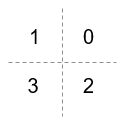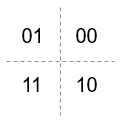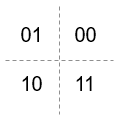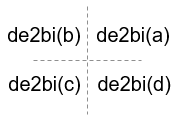# comm.OQPSKDemodulator

Demodulation using OQPSK method

## Description

The comm.OQPSKDemodulator object applies pulse shape filtering to the input waveform and demodulates it using the offset quadrature phase shift keying (OQPSK) method. For more information, see Pulse Shaping Filter. The input is a baseband representation of the modulated signal.

For information about delays incurred by modulator-demodulator pair processing, see Modulation Delays.

To demodulate a signal that is OQPSK modulated:

1. Create the `comm.OQPSKDemodulator` object and set its properties.

2. Call the object with arguments, as if it were a function.

## Creation

### Syntax

``oqpskdemod = comm.OQPSKDemodulator``
``oqpskdemod = comm.OQPSKDemodulator(mod)``
``oqpskdemod = comm.OQPSKDemodulator(Name,Value)``
``oqpskdemod = comm.OQPSKDemodulator(phase,Name,Value)``

### Description

````oqpskdemod = comm.OQPSKDemodulator` creates a demodulator System object™. This object can jointly match-filter and decimate a waveform, and demodulate it using the offset quadrature phase shift keying (OQPSK) method.```
````oqpskdemod = comm.OQPSKDemodulator(mod)` creates a demodulator System object with symmetric configuration to the OQPSK modulator object, `mod`.```

example

````oqpskdemod = comm.OQPSKDemodulator(Name,Value)` sets properties using one or more name-value pairs. Enclose each property name in single quotes.Example: `comm.OQPSKDemodulator('BitOutput',true)````
````oqpskdemod = comm.OQPSKDemodulator(phase,Name,Value)` sets the PhaseOffset property of the created object to `phase` and sets any other specified `Name`, `Value` pairs.Example: `comm.OQPSKDemodulator(0.5*pi,'SamplesPerSymbol',2)````

## Properties

expand all

Unless otherwise indicated, properties are nontunable, which means you cannot change their values after calling the object. Objects lock when you call them, and the `release` function unlocks them.

If a property is tunable, you can change its value at any time.

Phase offset from π/4, specified as a scalar in radians. The phase offset is applied to the zeroth point of the signal constellation before delay of quadrature component. After the OQPSK imaginary-component delay the signal is normalized with unity power.

Example: `'PhaseOffset',pi/4` aligns the zeroth point of the QPSK signal constellation point on the axes, {(1,0), (0,j), (-1,0), (0,-j)}.

Data Types: `double`

Option to output data as bits, specified as `false` or `true`.

• When you set this property to `false`, the object outputs a column vector of integer values with a length equal to the number of demodulated symbols. The output values are integer representations of two bits and range from `0` to `3`.

• When you set this property to `true`, the object outputs a binary column vector of bit values. The output vector length is twice as long as the number of input symbols.

Data Types: `logical`

Signal constellation bit mapping, specified as `'Gray'`, `'Binary'`, or a custom 4-element numeric vector of integers with values from 0 to 3.

SettingConstellation Mapping for IntegersConstellation Mapping for BitsComment

`Gray`The signal constellation mapping is Gray-encoded.

`Binary`The signal constellation mapping for the input integer `m` (0 ≤ `m` ≤ 3) is the complex value ```e(j*(PhaseOffset+π/4) + j*2*π*m/4)```.

Custom 4-element numeric vector of integers with values from 0 to 3Elements [a b c d] must be composed of the set of values [0, 1, 2, 3] in any order.

Data Types: `char` | `double`

Filtering pulse shape, specified as `'Half sine'`, ```'Normal raised cosine'``` | `'Root raised cosine'`, or `'Custom'`.

Data Types: `char`

Raised cosine filter rolloff factor, specified as a scalar from `0` to `1`.

#### Dependencies

This property is enabled when PulseShape is `'Normal raised cosine'` or ```'Root raised cosine'```.

Data Types: `double`

Filter length in symbols, specified as a scalar. An ideal raised cosine filter has an infinite impulse response. However, to realize a practical implementation of this filter, the object truncates the impulse response to `FilterSpanInSymbols` symbols.

#### Dependencies

This property is enabled when PulseShape is `'Normal raised cosine'` or ```'Root raised cosine'```.

Data Types: `double`

Filter numerator, specified as a row vector.

#### Dependencies

This property is enabled when PulseShape is `'Custom'`.

Data Types: `double`
Complex Number Support: Yes

Number of samples per symbol, specified as a positive even integer.

Data Types: `double`

Data type assigned to output, specified as `'double'`, `'single'`, or `'uint8'`.

Data Types: `char`

## Usage

### Syntax

``outsignal = oqpskdemod(waveform)``

### Description

example

````outsignal = oqpskdemod(waveform)` returns the demodulated output signal. The object produces one output symbol for each input pulse.```

### Input Arguments

expand all

Received waveform, specified as a complex scalar or column vector.

Data Types: `double`
Complex Number Support: Yes

### Output Arguments

expand all

Demodulated signal, returned as an NS-element integer vector or bit vector, where NS is the number of samples.

The received waveform is pulse shaped according to the configuration properties PulseShape and SamplesPerSymbol. The setting of the BitOutput property determines the interpretation of the received waveform.

Data Types: `double`

## Object Functions

To use an object function, specify the System object as the first input argument. For example, to release system resources of a System object named `obj`, use this syntax:

`release(obj)`

expand all

 `step` Run System object algorithm `release` Release resources and allow changes to System object property values and input characteristics `reset` Reset internal states of System object

## Examples

collapse all

Create an OQPSK modulator and demodulator pair. Create an AWGN channel object having two bits per symbol.

```oqpskmod = comm.OQPSKModulator('BitInput',true); oqpskdemod = comm.OQPSKDemodulator('BitOutput',true); channel = comm.AWGNChannel('EbNo',4,'BitsPerSymbol',2);```

Create an error rate calculator. To account for the delay between the modulator and demodulator, set the `ReceiveDelay` property to `2`.

`errorRate = comm.ErrorRate('ReceiveDelay',2);`

Process 300 frames of data looping through these steps.

• Generate vectors with 100 elements of random binary data.

• OQPSK-modulate the data. The data frames are processed as 50 sample frames of 2-bit binary data.

• Pass the modulated data through the AWGN channel.

• OQPSK-demodulate the data.

• Collect error statistics on the frames of data.

```for counter = 1:300 txData = randi([0 1],100,1); modSig = oqpskmod(txData); rxSig = channel(modSig); rxData = oqpskdemod(rxSig); errorStats = errorRate(txData,rxData); end```

Display the error statistics.

`ber = errorStats(1)`
```ber = 3.3336e-05 ```
`numErrors = errorStats(2)`
```numErrors = 1 ```
`numBits = errorStats(3)`
```numBits = 29998 ```

Perform OQPSK modulation and demodulation and apply root raised cosine filtering to a waveform.

System initialization

Define simulation parameters and create objects for OQPSK modulation and demodulation.

```sps = 12; % samples per symbol bits = randi([0, 1], 800, 1); % transmission data modulator = comm.OQPSKModulator('BitInput',true,'SamplesPerSymbol',sps,'PulseShape','Root raised cosine'); demodulator = comm.OQPSKDemodulator(modulator);```

Waveform transmission and reception

Use the `modulator` object to apply OQPSK modulation and transmit filtering to the input data.

```oqpskWaveform = modulator(bits); ```

Pass the waveform through a channel.

```snr = 0; rxWaveform = awgn(oqpskWaveform, snr);```

Use the `demodulator` object to apply receive filtering and OQPSK demodulation to the waveform.

```demodData = demodulator(rxWaveform); ```

Compute the bit error rate to confirm the quality of the data recovery.

```delay = (1+modulator.BitInput)*modulator.FilterSpanInSymbols; [~, ber] = biterr(bits(1:end-delay), demodData(delay+1:end))```
```ber = 0 ```

Use the `qamdemod` function to simulate soft decision output for OQPSK-modulated signals.

Generate an OQPSK modulated signal.

```sps = 4; msg = randi([0 1],1000,1); oqpskMod = comm.OQPSKModulator('SamplesPerSymbol',sps,'BitInput',true); oqpskSig = oqpskMod(msg);```

Add noise to the generated signal.

`impairedSig = awgn(oqpskSig,15);`

Perform Soft-Decision Demodulation

Create QPSK equivalent signal to align in-phase and quadrature.

`impairedQPSK = complex(real(impairedSig(1+sps/2:end-sps/2)), imag(impairedSig(sps+1:end)));`

Apply matched filtering to the received OQPSK signal.

```halfSinePulse = sin(0:pi/sps:(sps)*pi/sps); matchedFilter = dsp.FIRDecimator(sps,halfSinePulse,'DecimationOffset',sps/2); filteredQPSK = matchedFilter(impairedQPSK);```

To perform soft demodulation of the filtered OQPSK signal use the `qamdemod` function. Align symbol mapping of `qamdemod` with the symbol mapping used by the `comm.OQPSKModulator`, then demodulate the signal.

```oqpskModSymbolMapping = [1 3 0 2]; demodulated = qamdemod(filteredQPSK,4,oqpskModSymbolMapping,'OutputType','llr');```

expand all

## Extended Capabilities

### Topics

Introduced in R2012a